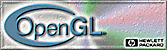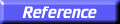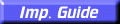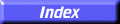# glGetClipPlane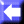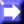glGetClipPlane: return the coefficients of the specified clipping plane.

C Specification | Parameters | Description | Notes | Errors | See Also

##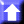C Specification

```void glGetClipPlane(
GLenum	 plane,
GLdouble	 *equation)
```

##Parameters

plane
Specifies a clipping plane. The number of clipping planes depends on the implementation, but at least six clipping planes are supported. They are identified by symbolic names of the form GL_CLIP_PLANEi where 0
i < GL_MAX_CLIP_PLANES.
equation
Returns four double-precision values that are the coefficients of the plane equation of plane in eye coordinates. The initial value is (0, 0, 0, 0).

##Description

glGetClipPlane returns in equation the four coefficients of the plane equation for plane.

##Notes

It is always the case that GL_CLIP_PLANEi = GL_CLIP_PLANE0 + i.

If an error is generated, no change is made to the contents of equation.

##Errors

• GL_INVALID_ENUM is generated if plane is not an accepted value.
• GL_INVALID_OPERATION is generated if glGetClipPlane is executed between the execution of glBegin and the corresponding execution of glEnd.

glClipPlane